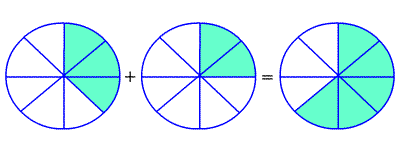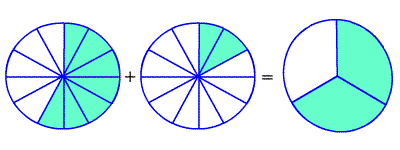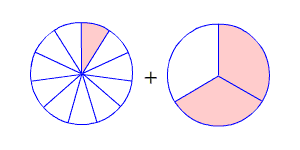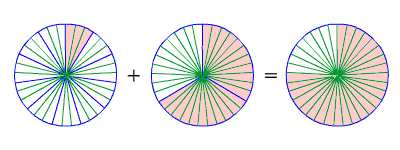When adding fractions, the first thing to check is if the denominators are the same.

## Adding Fractions with Like Denominators

Fractions with the same denominators are called like fractions.

To add fractions with like denominators, add the numerators , and write the sum over the denominator.

$\frac{3}{8}+\frac{2}{8}=\frac{5}{8}$Example :

Find $\frac{4}{9}+\frac{3}{9}$ .

Since the denominators are the same, add the numerators.

$=\frac{4+3}{9}$

$=\frac{7}{9}$

You may get an answer which is not in lowest terms , even if the fractions you were adding and subtracting both were. In this case, you have to reduce the fraction .

$\frac{7}{12}+\frac{1}{12}=\frac{8}{12}=\frac{8\text{\hspace{0.17em}}÷\text{\hspace{0.17em}}4}{12\text{\hspace{0.17em}}÷\text{\hspace{0.17em}}4}=\frac{2}{3}$## Adding Fractions with Unlike Denominators

If the denominators are not the same, then you have to use equivalent fractions which do have a common denominator . To do this, you need to find the least common multiple (LCM) of the two denominators.

For example, suppose you want to add:

$\frac{1}{11}+\frac{2}{3}$The LCM of $3$ and $11$ is $33$ . So, we need to find fractions equivalent to $\frac{1}{11}$ and $\frac{2}{3}$ which have $33$ in the denominator. Multiply the numerator and denominator of $\frac{1}{11}$ by $3$ , and multiply the numerator and denominator of $\frac{2}{3}$ by $11$ .

$\left(\frac{1\text{\hspace{0.17em}}×\text{\hspace{0.17em}}3}{11\text{\hspace{0.17em}}×\text{\hspace{0.17em}}3}\right)+\left(\frac{2\text{\hspace{0.17em}}×\text{\hspace{0.17em}}11}{3\text{\hspace{0.17em}}×\text{\hspace{0.17em}}11}\right)=\frac{3}{33}+\frac{22}{33}$

Now we have like denominators, and we can add as described above.

$=\frac{25}{33}$